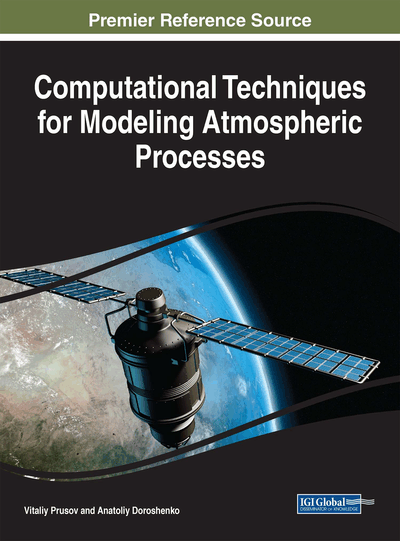# Methods of Mathematical Modeling Atmospheric Circulation

DOI: 10.4018/978-1-5225-2636-0.ch004
Available
\$37.50
No Current Special Offers

## Abstract

In this chapter, atmospheric processes are considered on a planetary scale that is impossible to imagine without a detailed three-dimensional modeling based on geophysical fluid dynamics equations of thermodynamics, radiation transfer, the kinetic equations describing the actual movement of air masses, conversion and transfer of energy and matter, the formation of clouds, aerosols, and rainfall and other atmospheric processes.
Chapter Preview
Top

## Planetary And Hemispheric Atmosphere Model: Isobaric Coordinate System

All the processes occurring in the Earth’s atmosphere and associated with the movement of air masses are inherently heterogeneous on their properties in the space and variability in time. Spatial scales are determined by the size or length of the disturbance wave, and the time scales – by the time of their existence, or the period of oscillation. The range of these phenomena varies significantly: from very small short-term turbulence to the jet stream (giant waves). Globally, these movements determine the transfer of momentum, heat, passive contaminants of water, dust, and minor constituents.

Unequal streams of solar radiation and the heterogeneity of the Earth’s surface in different geographical areas cause uneven heating of the air. This results in the movements in the atmosphere, which promote the redistribution of heat. The water vapor and impurities of various chemical substances also affect the processes in the atmosphere. Under certain conditions, the vapor condenses, creating a mist and clouds. Clouds are the source of many atmospheric phenomena – rain, thunderstorms, and a variety of optical effects. In addition, clouds significantly alter the energy resources in the atmosphere, because the extra heat is released during condensation of water vapor and clouds themselves sensitively reduce the influx of solar radiation to the Earth’s surface and reduce its heat loss. Although the amount of internal (potential) energy transformed into kinetic energy is relatively small, this process has a significant impact on the energy of the atmospheric circulation. The manifestation of this energy is felt in the convective zones, jet streams, winds associated with the trough, and others. They are subject to weather forecasts. A small amount of the energy converts shows a subtle imbalance, whereby kinetic energy is generated, what also affects to the complexity of theoretical studies of such processes.

Let us recall and analyze the system of equations (3.2.29) – (3.2.35) with respect to the modeling of large-scale atmospheric circulation. Since in the large-scale atmospheric processes vertical acceleration is negligibly small, the equation (3.2.30), which expresses the law of conservation of momentum, is only used to describe the horizontal movement, and the equation of motion in the projection on the vertical axis, is simply reduced to the hydrostatic equation

. (4.1.1)

This equation is the fundamental relation that connects the height z and the pressure p. The validity of (4.1.1) for the Earth’s atmosphere is confirmed by both theoretical and field observations. It was found that in the process of adapting the atmosphere is always committed to the state of hydrostatic equilibrium. This feature and the need to address the underlying surface orography make appropriate to use the pressure p as the independent variable instead of height z. When constructing barometric topography maps, hydrometeorological services adopt geopotential of the isobaric surface Φ=gz as the function in question, so this dictates the transition to the isobaric coordinates. The pressure p in this case plays the role of the independent variable.

Before proceeding to adapt the system of equations (3.2.29) – (3.2.35) to the isobaric coordinate system, we must agree that the orographic field hydrodynamic model (3.2.29) – (3.2.35) has the same horizontal resolution as meteorological fields that are included in the model. Therefore, as the field of orography is a function of the horizontal coordinates, we a priori leave aside the issue of the horizontal finite-difference approximation errors influence on the pressure gradient in the case of orographic sub-grid scale, i.e. when the orographic field is due to the very steep mountains.

The velocity field V = (u,v,ϖ) at an isobaric coordinate system is a rate of change of isobaric coordinates ofan elemental air volume

and allows obtaining the equations of motion for the variables (u,v,ϖ). To do this, we transform the full (the substantial) derivative (3.3.1), considering the transition from the old variables (x,y,z) to the new variables (x,y,p), (4.1.2) where ϖ=dp/dt is the vertical velocity.

## Complete Chapter List

Search this Book:
Reset